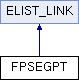Tesseract  3.02
FPSEGPT Class Reference

`#include <pitsync1.h>`

Inheritance diagram for FPSEGPT:List of all members.

## Public Member Functions

FPSEGPT ()
FPSEGPT (inT16 x)
FPSEGPT (inT16 x, BOOL8 faking, inT16 offset, inT16 region_index, inT16 pitch, inT16 pitch_error, FPSEGPT_LIST *prev_list)
FPSEGPT (FPCUTPT *cutpt)
inT32 position ()
double cost_function ()
double squares ()
double sum ()
FPSEGPTprevious ()
inT16 cheap_cuts () const

BOOL8 faked
BOOL8 terminal
inT16 fake_count

## Detailed Description

Definition at line 34 of file pitsync1.h.

## Constructor & Destructor Documentation

 FPSEGPT::FPSEGPT ( ) ` [inline]`

Definition at line 37 of file pitsync1.h.

```              {  //empty
}
```
 FPSEGPT::FPSEGPT ( inT16 x )

Definition at line 71 of file pitsync1.cpp.

``` :xpos (x) {
pred = NULL;
mean_sum = 0;
sq_sum = 0;
cost = 0;
faked = FALSE;
terminal = FALSE;
fake_count = 0;
mid_cuts = 0;
}
```
 FPSEGPT::FPSEGPT ( inT16 x, BOOL8 faking, inT16 offset, inT16 region_index, inT16 pitch, inT16 pitch_error, FPSEGPT_LIST * prev_list )

Definition at line 91 of file pitsync1.cpp.

``` :xpos (x) {
inT16 best_fake;               //on previous
FPSEGPT *segpt;                //segment point
inT32 dist;                    //from prev segment
double sq_dist;                //squared distance
double mean;                   //mean pitch
double total;                  //total dists
double factor;                 //cost function
FPSEGPT_IT pred_it = prev_list;//for previuos segment

cost = MAX_FLOAT32;
pred = NULL;
faked = faking;
terminal = FALSE;
best_fake = MAX_INT16;
mid_cuts = 0;
for (pred_it.mark_cycle_pt (); !pred_it.cycled_list (); pred_it.forward ()) {
segpt = pred_it.data ();
if (segpt->fake_count < best_fake)
best_fake = segpt->fake_count;
dist = x - segpt->xpos;
if (dist >= pitch - pitch_error && dist <= pitch + pitch_error
&& !segpt->terminal) {
total = segpt->mean_sum + dist;
sq_dist = dist * dist + segpt->sq_sum + offset * offset;
//sum of squarees
mean = total / region_index;
factor = mean - pitch;
factor *= factor;
factor += sq_dist / (region_index) - mean * mean;
if (factor < cost) {
cost = factor;           //find least cost
pred = segpt;            //save path
mean_sum = total;
sq_sum = sq_dist;
fake_count = segpt->fake_count + faked;
}
}
}
if (fake_count > best_fake + 1)
pred = NULL;                 //fail it
}
```
 FPSEGPT::FPSEGPT ( FPCUTPT * cutpt )

Definition at line 50 of file pitsync1.cpp.

```                  {
pred = NULL;
mean_sum = cutpt->sum ();
sq_sum = cutpt->squares ();
cost = cutpt->cost_function ();
faked = cutpt->faked;
terminal = cutpt->terminal;
fake_count = cutpt->fake_count;
xpos = cutpt->position ();
mid_cuts = cutpt->cheap_cuts ();
}
```

## Member Function Documentation

 inT16 FPSEGPT::cheap_cuts ( ) const` [inline]`

Definition at line 66 of file pitsync1.h.

```                             {  //no of cheap cuts
return mid_cuts;
}
```
 double FPSEGPT::cost_function ( ) ` [inline]`

Definition at line 54 of file pitsync1.h.

```                           {
return cost;
}
```
 inT32 FPSEGPT::position ( ) ` [inline]`

Definition at line 51 of file pitsync1.h.

```                     {  //acces func
return xpos;
}
```
 FPSEGPT* FPSEGPT::previous ( ) ` [inline]`

Definition at line 63 of file pitsync1.h.

```                        {
return pred;
}
```
 double FPSEGPT::squares ( ) ` [inline]`

Definition at line 57 of file pitsync1.h.

```                     {
return sq_sum;
}
```
 double FPSEGPT::sum ( ) ` [inline]`

Definition at line 60 of file pitsync1.h.

```                 {
return mean_sum;
}
```

## Member Data Documentation

Definition at line 73 of file pitsync1.h.

Definition at line 71 of file pitsync1.h.

Definition at line 72 of file pitsync1.h.

The documentation for this class was generated from the following files: